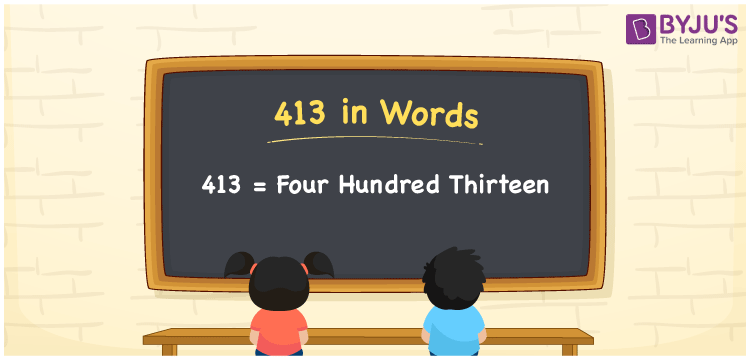# 413 in Words

413 in words is written as Four hundred thirteen. In the International System of Numerals and the Indian System of Numerals, 413 is written as Four hundred thirteen. The number 413 is a Cardinal Number as it represents some quantity. For example, “this reaction takes place at 413K temperature”. 413 represents “how much temperature”.

 413 in Words Four hundred thirteen Four hundred thirteen in Number 413

## 413 in English Words

We write 413 in English Words using the letters of the English alphabet. Therefore, we read 413 in English as “Four hundred thirteen.”## How to Write 413 in Words?

To write 413 in words, we shall use the place value chart. In the place value chart, write 4 in the hundreds, 1 in the tens, and 3 in the ones, respectively. Now let us make a place value chart to write the number 413 in words.

 Hundreds Tens Ones 4 1 3

Thus, we can write the expanded form as

4 × Hundred + 1 × Ten + 3 × One

= 4 × 100 + 1 × 10 + 3 × 1

= 400 + 10 + 3

= 413

= Four hundred thirteen.

413 is a natural number, the successor of 412 and the predecessor of 414.

413 in words – Four hundred thirteen

• Is 413 an odd number? – Yes
• Is 413 an even number? – No
• Is 413 a perfect square number? – No
• Is 413 a perfect cube number? – No
• Is 413 a prime number? – No
• Is 413 a composite number? – Yes

## Frequently Asked Questions on 413 in Words

Q1

### How to write 413 in words?

413 in words is written as Four hundred thirteen.
Q2

### How to write 413 in the International and Indian System of Numerals?

In both the system of numerals, 413 in words, is written as Four hundred thirteen.
Q3

### What are the factors of 413?

The factors of 413 are 1, 7, 59 and 413.